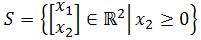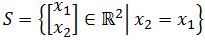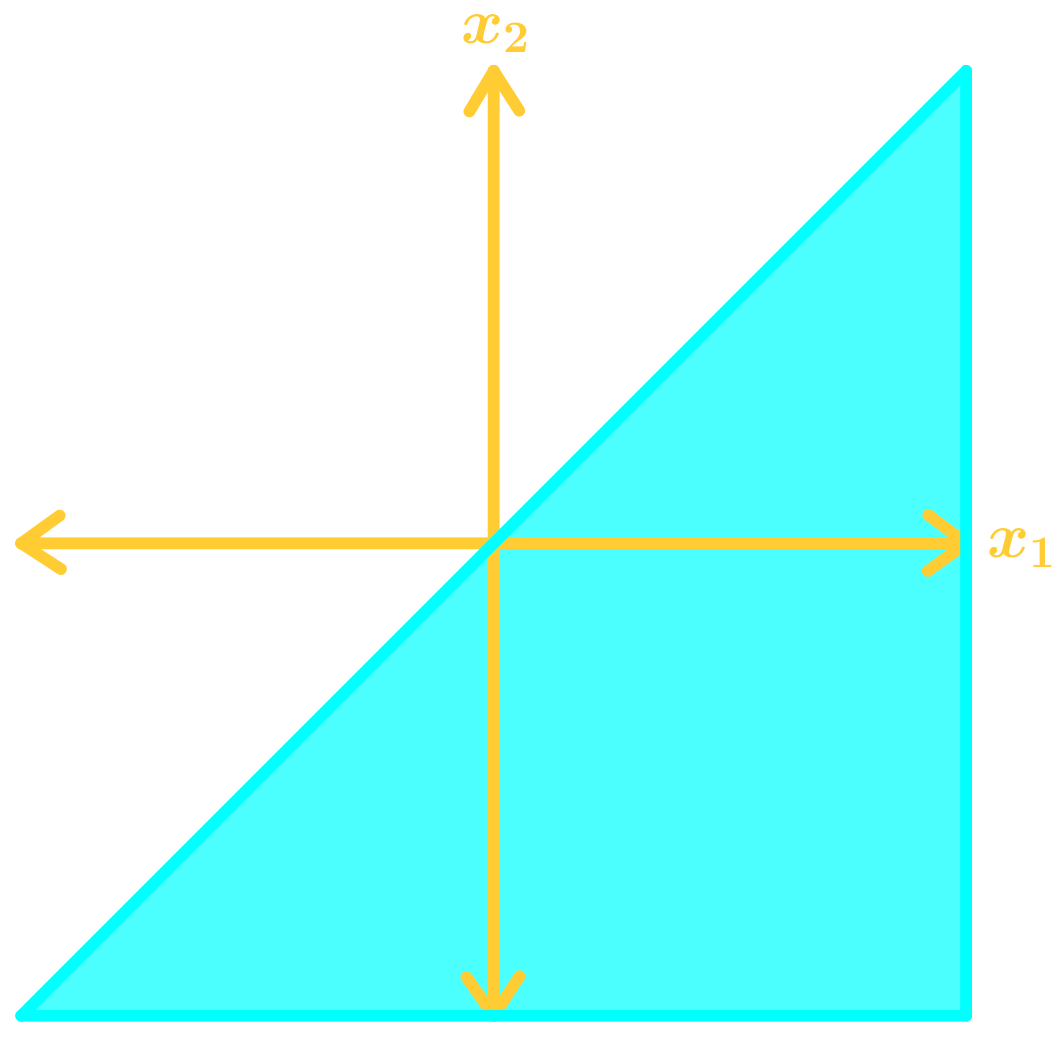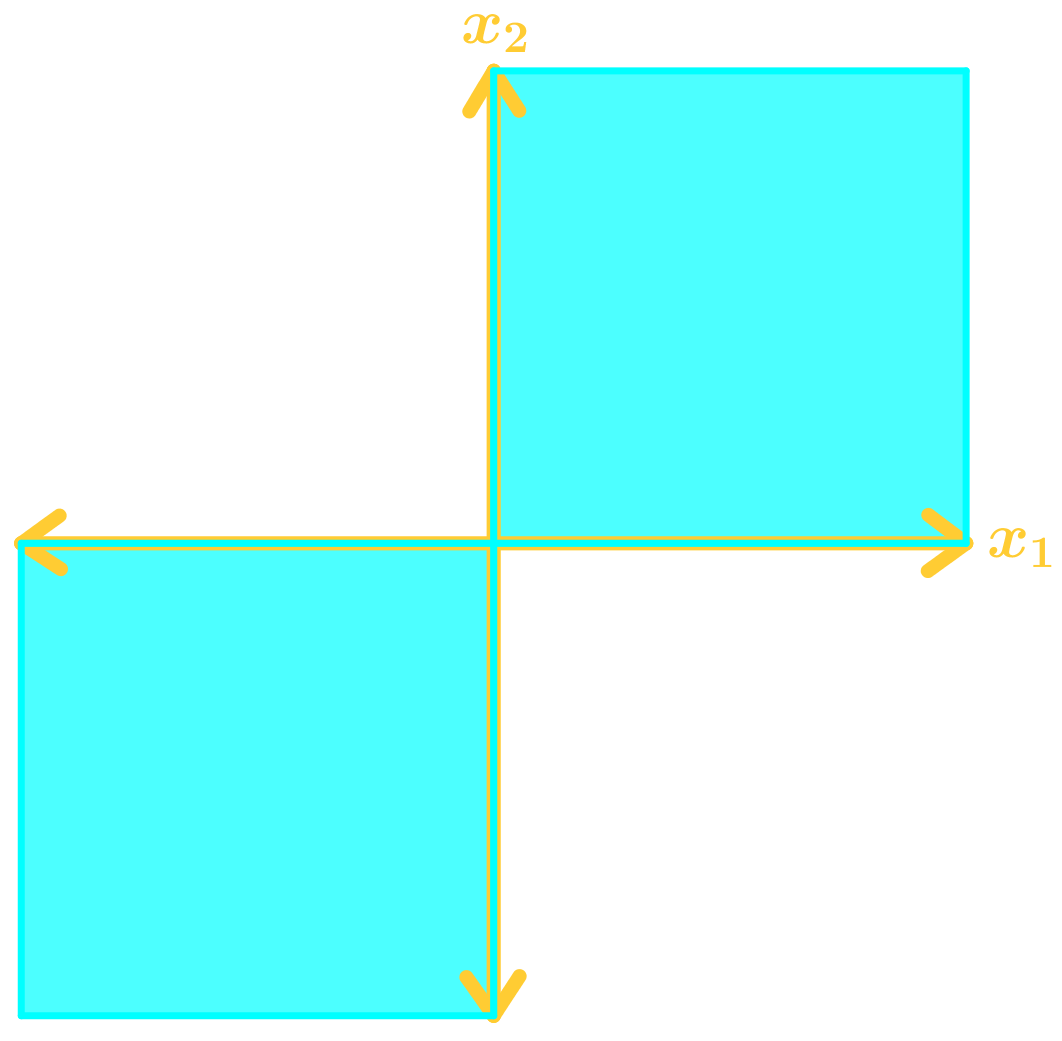# Properties of subspace

0/2
##### Introduction
###### Lessons
1. Properties of Subspace Overview:
2. A Subspace in $\Bbb{R}^n$
• The zero vector
• Closed under scalar multiplication
• Example of a Subspace
• Example of not a Subspace
3. Subspace of a span of vectors in $\Bbb{R}^n$
• Remember span = linear combination
• Showing a span of vectors is a subspace in $\Bbb{R}^n$
0/5
##### Examples
###### Lessons
1. Showing that a set is a subspace of $\Bbb{R}^n$
Is the following set a subspace of $\Bbb{R}^2$?1. Is the following set a subspace of $\Bbb{R}^2$?1. Showing that a set is a subspace of $\Bbb{R}^n$ with graphs
The following graph displays a set in $\Bbb{R}^2$. Assume the set includes the bounding lines. Give a reason as to why the set $S$ is not a subspace of $\Bbb{R}^2$.1. Showing that a set is a subspace of $\Bbb{R}^n$ with graphs
The following graph displays a set in $\Bbb{R}^2$. Assume the set includes the bounding lines. Give a reason as to why the set $S$ is not a subspace of $\Bbb{R}^2$.1. Showing a set equal to a span of vectors is a subspace of $\Bbb{R}^n$
Let $U=$ Span{$v_1,v_2,v_3$}, where $U$ is a set. Determine if $U$ is in the subspace of $\Bbb{R}^3$.
###### Free to Join!
StudyPug is a learning help platform covering math and science from grade 4 all the way to second year university. Our video tutorials, unlimited practice problems, and step-by-step explanations provide you or your child with all the help you need to master concepts. On top of that, it's fun - with achievements, customizable avatars, and awards to keep you motivated.
• #### Easily See Your ProgressWe track the progress you've made on a topic so you know what you've done. From the course view you can easily see what topics have what and the progress you've made on them. Fill the rings to completely master that section or mouse over the icon to see more details.
• #### Make Use of Our Learning Aids###### Practice Accuracy

See how well your practice sessions are going over time.

Stay on track with our daily recommendations.

• #### Earn Achievements as You LearnMake the most of your time as you use StudyPug to help you achieve your goals. Earn fun little badges the more you watch, practice, and use our service.
• #### Create and Customize Your AvatarPlay with our fun little avatar builder to create and customize your own avatar on StudyPug. Choose your face, eye colour, hair colour and style, and background. Unlock more options the more you use StudyPug.

### Properties of Subspace

The first thing we have to do in order to comprehend the concepts of subspaces in linear algebra is to completely understand the concept of $R^{n}$, or what is called: the real coordinate space of n-dimensions. For that, there are some basic terms you have to at least have a grasp of, such as: variables, dimension and coordinate space. Let us clarify these terms first in the next paragraphs.

We have used the word 'variable' already for many lessons, in or out of this course, the concept of a variable is used throughout all of mathematics. For linear algebra, when talking about vectors, we know a variable defines the direction of one of the components in a vector according to the geometric coordinate planes. Any coefficient associated with a variable defines the magnitude of that particular vector's component in the direction of that variable.

For example: If we are to have a vector $\overline{v}$ with three different variables, which we define to be in the direction of the 3-dimensional geometric space coordinates $\hat{x}$, $\hat{y}$,$\hat{z}$:

Notice that we are used, so far, to say a vector has variables x, y and z, but this time we have named them: $\hat{x}, \hat{y}, \hat{z}$. The reason for it is that we are giving these variables a graphic meaning, they represent the cartesian coordinate planes possible in three dimensions, which means we can graph this vector in a manner similar to:

Thus, we say vector $\overline{v}$ has three components, one in x, one in y and one in z. In other words, the $\overline{v}$ vector has a component with a certain magnitude in the direction of the x-plane, which is $c_{1}\hat{x}$; it has one component with a certain magnitude in the direction of the y-plane, which is $c_{2}\hat{y}$; and it has one component with a certain magnitude in the direction of the z-plane, which is $c_{3}\hat{z}$. The magnitudes corresponding to the component in each direction are defined by the coefficients $c_{1}, c_{2}, c_{3}$.

This takes us to the second concept which is dimensions. During this course we have used the word dimensions to talk about the size of a matrix (the number of rows and columns it contains) and although these are related in a certain way (which can be explained in other lessons), the word dimension is used differently here.

When talking about the dimensions of a vector we are truly talking about the dimensions needed to describe this particular vector, in other words, the coordinate planes (such as x, y and z) necessary to describe every single component contained in the vector. These, are at the same time, translated graphically as space dimensions which you can think of as: length, width, and depth, the three dimensions of space we live in; or even geographical dimensions: north-south, east-west, up-down.

For example, if we use a vector to describe the displacement of a car as it travels from one city to the next, we have to use a vector with three dimensions because our world (and the known universe, for that matter) has three dimensions of space: length, width and depth/height. Thus, if we want to completely describe the displacement of a car traveling from one city to the next with a vector, such vector MUST contain three components which in geographical coordinates would be: how much north or south it traveled, how much east or west it traveled, and how much change in altitude of the terrain it had (did it go up in the mountains? Or did it lose altitude by going to a valley? etc). Look at this example on how we can easily make a vector describing the displacement of a car from Stanley Park in Vancouver (which is at sea level) to Grouse Mountain nearby (with an altitude of 1,231 meters):

In figure 2 we could easily think on the east-to-west axis as the x-axis, the north-to-south axis as the y-axis, and the altitude axis as the positive z-axis and thus, notate this blue displacement vector with components in $\hat{x}, \hat{y}, \hat{z}$ (which would be the variables) where their coefficients $c_{1}, c_{2}, c_{3}$ would be the amount of meters on each direction necessary to arrive from Stanley Park, to Grouse Mountain. And so, we have a vector in three-dimensions of space: how much it went to the east, how much it went north and how much it went up in altitude.

And so, a coordinate space is that 'space' in itself, that 'stage' if you will, where things happen. The coordinate space will be the space which you are using as reference frame for the problem you are studying or solving. For example, in figure 2 for the vector displacement of a car from Stanley Park to Grouse Mountain we needed a coordinate space with three dimensions in order to completely describe the displacement asked, if we were to be looking at the displacement of a pencil in a piece of paper as you draw a line, there is only a need for two coordinate axes to describe that motion in two dimensions only, and so our coordinate space would have two dimensions; hence, depending the case in study, will be the coordinate space needed.

This is how we finally arrive to the concept of the real coordinate space of n-dimensions or simply called $R^{n}$. For any given scenario, $R^{n}$ provides the coordinate space where all of the components in a vector can be noted and represented. In other words, if we have a vector with two components in two different dimensions, then we say n=2 and the coordinate space needed to fully represent such vector is $R^{n}=R^{2}$. If in the other hand we have a vector with three different components in three different dimensions, then n=3 and $R^{n}=R^{3}$.

$R^{n}$ is important because it allows us to have a notation to represent cases in which the dimensions are more than three. If you think about it, we can only graphically represent vectors with up to three dimensions, that is, because we do not really have a way to know how a 4th dimension would look like since in our own universe, we cannot see a fourth dimension (physicists call it time, but, can you draw time?). If you go further than 4 dimensions, we could not even describe a universe with such a structure, it is not possible in our conception of the universe we live in (there are many theories, supported by mathematical concepts, but we always get to the issue of we cannot represent it graphically as it could exist, because if it does exist it is completely invisible not only to our eyes but to any senses and capacities). But again, mathematically speaking, there is an infinitely amount of dimensions that can be attributed to a vector and we can work the math of them.

At this point you may be wondering: Why would we want to work with vectors representing more than 3 different variable components which we associates with 3 different dimensions that we have no capacity to sense? Because in physical problems we can think of them not as dimensions in a vector, but just as simple different variables (the math is the same), and so, a vector having multiplica different variables does not always means each variable has a different dimension, they can share the way they displace to represent different physical properties of an scenario. In this case, Rngives us the 'stage', the space, the reference frame in which all of these variables occur.

### What is a vector subspace?

After such an extensive introduction about $R^{n}$, let us look at the main concept of today which is a vector subspace.

Simply said a subspace of a vector space (usually referred as vector subspace or simply, subspace) is a set of vectors in a particular $R^{n}$(which will have as many dimensions as are necessary depending on the components of the vectors in the set) that follows three conditions.

Remember that a set is just a list of elements, if we are talking about a set of vectors, then this means a subspace is a list of vectors, and thus, any subspace of $R^{n}$ is any set S in $R^{n}$ which has the next three properties:

1. Zero vector property
This property states that any set of vectors considered as a subspace in $R^{n}$ will contain the zero vector. Remember that the zero vector is that which has only zeros as components, therefore this rule entails that for a set of vectors to be considered a subspace in $R^{n}$ it needs to contain the origin (as in the origin of the cartesian coordinate system) when graphed.
This property states that for any vector u and v in a set, the sum of u+v is still part of the set S in order for this set to be considered as a subspace in $R^{n}$. In other words, if two vectors belonging to a given set are found in the subspace $R^{n}$, then the vector resulting from the addition of those two vectors must fall into $R^{n}$ too; if they happen to fall outside of the subspace $R^{n}$, then it means this whole set is NOT a subspace of $R^{n}$.
3. Closed under scalar multiplication property
The closed under scalar multiplication property means that for every vector belonging to a set S, in order for this set to be considered a subspace of $R^{n}$ it means that you can multiply any scalar to these vectors and the resulting vectors will still fall into the subspace $R^{n}$. In formal words, the property says: for each vector u in the set S, the vector cu (where c is a scalar) is in S.
With all of this in mind, a set of vectors which is a subspace in $R^{n}$ is defined as:
Looking at the definition of what is a subspace of a vector space, something interesting comes to mind: if we think on vector spaces and subspaces, a vector space is also a subspace of itself. And so, when comparing a vector space vs subspace, we realize that the main difference between vector space and subspace is just that the vector space is the one with the higher dimensions. Therefore, subspaces of vector spaces are selected parts of vector spaces with certain conditions attached to them, depending on the context.

### Vector subspace examples

Example 1

Is the following set a subspace of $R^{2}$?

In order to know if the set belongs to the subspace, we first graph the subspace according to the conditions provided in equation 3:

Now let us check if the three properties of a subspace of this R2 are met:

• Is there a zero vector?
Yes, the zero vector exists on this two dimensional plane delimited by $x_{2} \leq 0$. This is because the zero vector is the origin, in two dimensions this means that:
Therefore in this two dimensional plane where $x_{1}$ can have any value, (and thus, a value of zero is possible) and $x_{2} \leq 0$ (where a value of zero is also possible) this first property condition is met.
• Is the set closed under addition?
In order to check for this property, we define two vectors as:
Where the components for $x_{1}(a and c)$ can be anything and the components of $x_{2}$ both meet the condition established in the problem since both $b \leq 0$ and $d \leq 0$. Therefore, if we add those two general vectors we obtain:
Where $b+d \leq 0$ and so, this set is closed under addition.
• Is the set closed under scalar multiplication?
To check for the property of closeness under scalar multiplication means to check that every single vector in the space can be multiplied by any scalar (any real number) and still produce a result inside the vector space. In this case, if we were to have any vector inside the provided space multiplied by a negative scalar, this would produce a new vector with negative components in the both axes. In the case of the component of $x_{1}$ this wouldn't be an issue since you can have any value there, but for the component in $x_{2}$ you cannot have negative values, and so, this set of vectors is not closed under scalar multiplication.
Example 2

The following graph displays a set in $R^{2}$. Assume the set includes the bounding lines. Give reason as to why the set S is not a subspace of $R^{2}$.

Now let us check if the three properties of a subspace of this $R^{2}$ are met:

• Is there a zero vector?
Yes, the origin is inside the shaded area on the graph, therefore the vector space contains the zero vector.
• Is the set closed under addition?
Yes, this vector set is closed under addition because when any two vectors in the set are added to each other, they produce another vector that will be located inside the vector space too.
• Is the set closed under scalar multiplication?
The set is not closed under scalar multiplication because when any of the vectors in the set gets multiplied by a negative scalar, this multiplication changes the direction of the vector to its opposite one, and all of those are out of the vector space.
The effects of the three properties in the vectors from the set can be observed in the next figure: Whenever you think on what would be the method on how to determine if a vector is in a subspace, this graphic representations are usually the best to work with.
Conclusion: the set S is not a subspace of R2 because it does not meet the property of cloneness under scalar multiplication.
Example 3
The following graph displays a set in $R^{2}$. Assume the set includes the bounding lines. Give reason as to why the set S is not a subspace of $R^{2}$.
In order to determine whether the given set S is a subspace of the vector space we check if the three properties of a subspace of this $R^{2}$ are met:
• Is there a zero vector?
Yes, the origin is inside the shaded area on the graph, therefore the vector space contains the zero vector.
• Is the set closed under addition?
No, when added with one another not all of the vectors in the set produce a vector still inside of the vector space, therefore, this property is not met.
• Is the set closed under scalar multiplication?
There is no need to check for this property anymore since the second one wasn't met.
The effects of the two properties in the vectors from the set can be observed in the next figure:
Conclusion: the set S is not a subspace of $R^{2}$ because it does not meet the property of cloneness under addition.
A subspace of $\Bbb{R}^n$ is any set $S$ in $\Bbb{R}^n$ that has the three following properties:
1) The zero vector is in $S$
2) For each $u$ and $v$ in the set $S$, the sum of $u+v$ is in $S$ (closed under addition)
3) For each $u$ in the set $S$, the vector $cu$ is in $S$. (closed under scalar multiplication)